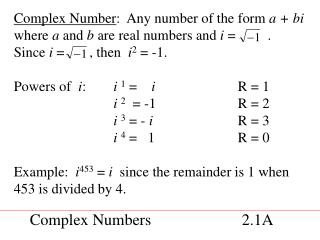DownloadDownload PresentationComplex Numbers 2.1A

# Complex Numbers 2.1A

Download Presentation## Complex Numbers 2.1A

- - - - - - - - - - - - - - - - - - - - - - - - - - - E N D - - - - - - - - - - - - - - - - - - - - - - - - - - -
##### Presentation Transcript

1. Complex Number: Any number of the form a + bi where a and b are real numbers and i = . Since i = , then i2 = -1. Powers of i: i1 = i R = 1 i2 = -1 R = 2 i3 = - i R = 3 i4 = 1 R = 0 Example: i453 = isince the remainder is 1 when 453 is divided by 4. Complex Numbers 2.1A

2. Addition: (2 + 5i) + (3 – 4i) = (2 + 3) + (5i – 4i) = 5 +i Subtraction: (2 + 5i) – (3 – 4i) = (2 + 5i) + (-3 + 4i) = (2 + -3) + (5i + 4i) = -1 + 9i Multiplication: (2 + 5i) (3 – 4i) = 6 – 8i +15i – 20i2 = 6 + 7i – 20(-1) = 26 + 7i Operations on Complex Numbers 2.2A

3. To divide complex numbers, multiply numerator and denominator by the conjugateof the denominator. The conjugate of a + bi is a – bi. Dividing Complex Numbers 2.3A

4. Complex Plane Imaginary axis A: 3 + 2i B: 4i C: -2 – i Real axis Absolute value of a + bi : Complex Plane/Absolute Value of Complex Number 2.4A